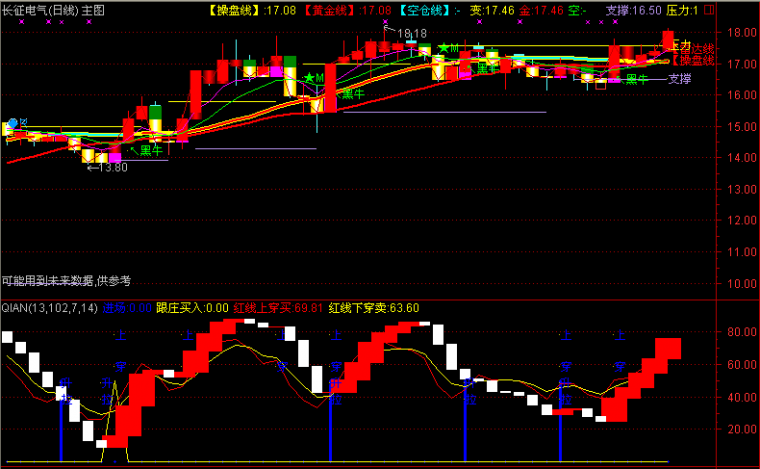﻿ 三金叉见底指标公式（代编写程序化交易模型）-同花顺公式 －程序化交易（CXH99.COM）

# 三金叉见底指标公式[同花顺公式]

1赋值:[变量名错误]成交量(手)的5日简单移动平均

2赋值:[变量名错误]成交量(手)的10日简单移动平均

1赋值:[变量名错误]收盘价的5日简单移动平均

2赋值:[变量名错误]收盘价的10日简单移动平均

IF (k<=0)RETURN 0;MAVOL\$1:=MA(VOL,5);IF (k<=1)RETURN 0;MAVOL\$2:=MA(VOL,10);DIFF : =EMA(CLOSE,12) - EMA(CLOSE,26);DEA : =EMA(DIFF,9);MACD : =2*(DIFF-DEA);IF (k<=0)RETURN 0;MA\$1:=MA(CLOSE,5);IF (k<=1)RETURN 0;MA\$2:=MA(CLOSE, 10);二金叉1:IF((CROSS(MAVOL\$1,MAVOL\$2) AND CROSS(MA\$1,MA\$2)),1,0);二金叉2:IF((CROSS(MACD,0) AND CROSS(MA\$1,MA\$2)),2,0);二金叉3:IF((CROSS(MACD,0) AND CROSS(MAVOL\$1,MAVOL\$2)),3,0);三金叉:IF((CROSS(MACD,0) AND CROSS(MAVOL\$1,MAVOL\$2) AND CROSS(MA\$1,MA\$2)),5,0);量比:VOL/REF(VOL,2)-1;【字体： 】【打印文章】【查看评论

没有相关内容